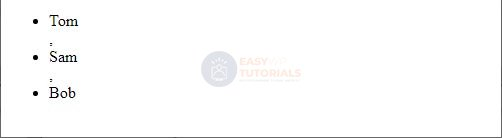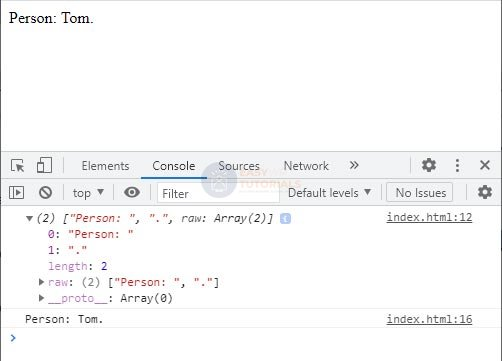# JavaScript Row patterns

String templates (template strings/template literals) allow you to insert different values ​​into a string. This technique is also called interpolation. To do this, the strings are enclosed in slash quotes, and the value to be inserted is preceded by the \$ symbol and enclosed in curly braces:

```const name = "Tom";
const hello = `Hello \${name}`;
console log(hello); // Hello Tom
```

Here, the \${name}value of the constant will be inserted in place name. Thus, from the template `Hello \${name}`we will get the string Hello Tom.

Similarly, several values ​​can be inserted into a string at once:

```const name = "Tom";
let age = 37;
const userInfo = `\${name} is \${age} years old`;
console.log(userInfo); // Tom is 37 years old
```

Also, instead of scalar values, properties of complex objects can be added:

```const tom ={
name: "Tom",
age: 22
}
const tomInfo = `\${tom.name} is \${tom.age} years old`;
console log(tominfo); // Tom is 22 years old
```

You can also insert more complex calculated expressions:

```function sum(x, y){
return x + y;
}
let a = 5;
let b = 4;

const result = `\${a} + \${b} = \${sum(a, b)}`;
console log(result); // 5 + 4 = 9

let expression = `\${a} * \${b} = \${ a * b}`;
console log(expression); // 5 * 4 = 20
```

In the first case, a function is called in the template sum(), the parameters of which are passed the values ​​of the variables aand b: \${sum(a, b)}. As a result, the sum of a and b will be inserted into this place.

In the second case, the operation of multiplying variables is performed in the template: \${ a * b}.

### html code in templates

Templates can also store html code that will be dynamically generated.

```<!DOCTYPE html>
<html lang="en">
<meta charset="UTF-8">
<meta http-equiv="X-UA-Compatible" content="IE=edge">
<meta name="viewport" content="width=device-width, initial-scale=1.0">
<title>easywptutorials.com</title>
<body>
<script>
const tom = {name: "Tom", age: 37};
const markup = `<div>
<p><b>Name</b>: \${tom.name}</p>
<p><b>Age</b>: \${tom.age}</p>
</div>`;

document.body.innerHTML = markup;
</script>
</body>
</html>
```String templates in JavaScript

### Nested Templates

Let’s consider another example – let’s create an html list from the array elements:

```<!DOCTYPE html>
<html lang="en">
<meta charset="UTF-8">
<meta http-equiv="X-UA-Compatible" content="IE=edge">
<meta name="viewport" content="width=device-width, initial-scale=1.0">
<title>easywptutorials.com</title>
<body>
<script>
const people = [{name: "Tom", age: 37}, {name:"Sam", age: 41}, {name: "Bob", age: 21}];
const markup = `<ul>
\${people.map(person => `<li>\${person.name}</li>`)}
</ul>`;

document.body.innerHTML = markup;
</script>
</body>
</html>
```In this case, we are dealing with a nested template. That is, first a common external template is defined:

```const markup = `&lt;ul&gt;
\${.............}
&lt;/ul&gt;`;
```

And in a dynamically generated expression, another template is used:

```\${people.map(person =&gt; `&lt;li&gt;\${person.name}&lt;/li&gt;`)}
```

In this case, a function is called on the people array map(), which defines some action for each element of the array. This action is passed to map()as a function. Here, for simplicity, a lambda expression is used as such a function. It takes each element of the array as a parameter person and forms a string template for it `

```\${person.name}
```

### Passing a String Template to a Function

JavaScript allows you to pass a string template to a function, and not just as a string, but all of its dynamically calculated fragments as separate parameters. This feature can be used, for example, to preprocess templates and their values.

Consider the following example:

```<!DOCTYPE html>
<html lang="en">
<meta charset="UTF-8">
<meta http-equiv="X-UA-Compatible" content="IE=edge">
<meta name="viewport" content="width=device-width, initial-scale=1.0">
<title>easywptutorials.com</title>
<body>
<script>
const person = "Tom";

function check (parts, name){
console.log(parts);
return parts + name + parts;
}
let checkedTemplate = check`Person: \${person}.`;
console.log(checkedTemplate);

document.body.innerHTML = checkedTemplate;
</script>
</body>
</html>
``````function check (parts, name){
console.log(parts);
return parts + name + parts;
}
```

The parameter parts is an array of template parts separated by dynamic fragments to be inserted. The second parameter – name is a dynamically calculated template fragment. That is, in this case, we assume that the string template that is passed to the function check()will have only one dynamically calculated fragment. Accordingly, the array parts will have two elements: the static part of the template, which comes before the calculated fragment, and the part of the template, which comes after.

To make it clearer what we are talking about, in the function we output these elements of the parts array to the console.

The function returns return parts + name + parts, that is, in fact, we simply return the previously formed template without changing anything.

Notice how we pass a template to this function:

let checkedTemplate = check`Person: \${person}.`;

the pattern is simply specified after the function name.

From the console output, we see that the elements of the parts array are the substrings “Person: ” and “.”. And the namestring “Tom” is passed as the parameter value. It is worth noting that even if there were no more characters after the dynamically calculated fragment (for example, `Person: \${person}`), the parts array would still have two elements, only the second element would then be an empty string.

But in the example above, we simply returned the same content that was generated from the template. However, we can do some processing:

```const tom = "Tom";

function check (parts, name){
if(name === "Admin") return "User not defined";
else return parts + name + parts;
}
let checkedTemplate1 = check`User: \${tom}`;

console.log(checkedTemplate1);
console.log(checkedTemplate2);
```

In this case, if the value “Admin” is passed to the template, then we return one result, otherwise, we return what would have been formed based on the template.

Handling String Patterns in Functions in JavaScript

Templates with a large number of computed fragments can be handled in a similar way:

```const tom = {name: "Tom", age: 37};
const bob = {name: "Bob", age: 11};

function check (parts, name, age){
if(age &gt; 18) return `\${parts}\${name}. Access is open`;
else return `User \${name} has been denied access. Age \${age} is not valid`;
}
let checkedTemplate1 = check`User: \${tom.name} \${tom.age}`;
let checkedTemplate2 = check`User: \${bob.name} \${bob.age}`;

console.log(checkedTemplate1);
console.log(checkedTemplate2);
```

In this case, the template contains two dynamic fragments. Accordingly, the part array will have three elements.

In the function check(), depending on the value of the second dynamic frame (the conditional age of the user), we return one or another value.

Console output:

User: Tom. Access is open
User Bob has no access. Age 11 is invalid